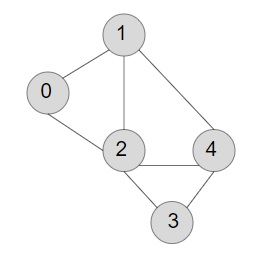# Count number of edges in an undirected graph in C++

C++Server Side ProgrammingProgramming

Given the task is to count the number of edges in an undirected graph. An undirected graph is a set of vertices which are connected together to form a graph, whose all the edges are bidirectional. Undirected graphs can travel in any direction from one node to another connected node.

Below is a visual representation of the undirected graph.Now, according to the problem we have to find the number of edges in the undirected graph.

Edges in a graph are the lines to which two vertices are joined.

Input

insert(graph_list, 0, 1);
insert(graph_list, 0, 2);
insert(graph_list, 1, 2);
insert(graph_list, 1, 4);
insert(graph_list, 2, 4);
insert(graph_list, 2, 3);
insert(graph_list, 3, 4);

Output

count of edges are: 7

## Approach we will be opting to solve the above problem −

• Initialise a list to store all the vertices of the graph’s list and insert the values accordingly.

• In function count_edges, declare a variable count=0 which is to return the count of the edges.

• Traverse the list using a loop until we reach the last vertice and add the value of count with graph_list[i].size() and store it back to the count variable.

• After we reach the last vertice, divide the value of count by two, and print the result.

## Example

Live Demo

#include<bits/stdc++.h>
using namespace std;
//function to insert vertices
void insert(list<int> graph_list[], int u, int v){
graph_list[u].push_back(v);
graph_list[v].push_back(u);
}
//function to count the total number of edges
void count_edges(list<int> graph_list[], int v){
int count=0;
//traverse the loop till the vertice is found
for (int i = 0 ; i < v ; i++){
count += graph_list[i].size();
}
count = count/2;
cout<<"count of edges are: "<<count;
}
int main(int argc, char* argv[]){
//creating 5 vertices in a graph
int vertices = 5;
//declare list to create a graph and pass the vertices
list<int> graph_list[vertices];
//call insert function passing the list variable, vertice, linked vertice
insert(graph_list, 0, 1);
insert(graph_list, 0, 2);
insert(graph_list, 1, 2);
insert(graph_list, 1, 4);
insert(graph_list, 2, 4);
insert(graph_list, 2, 3);
insert(graph_list, 3, 4);
//calling count function that will count the edges
count_edges(graph_list, vertices);
return 0 ;
}

## Output

If we run the above code we will get the following output −

count of edges are: 7